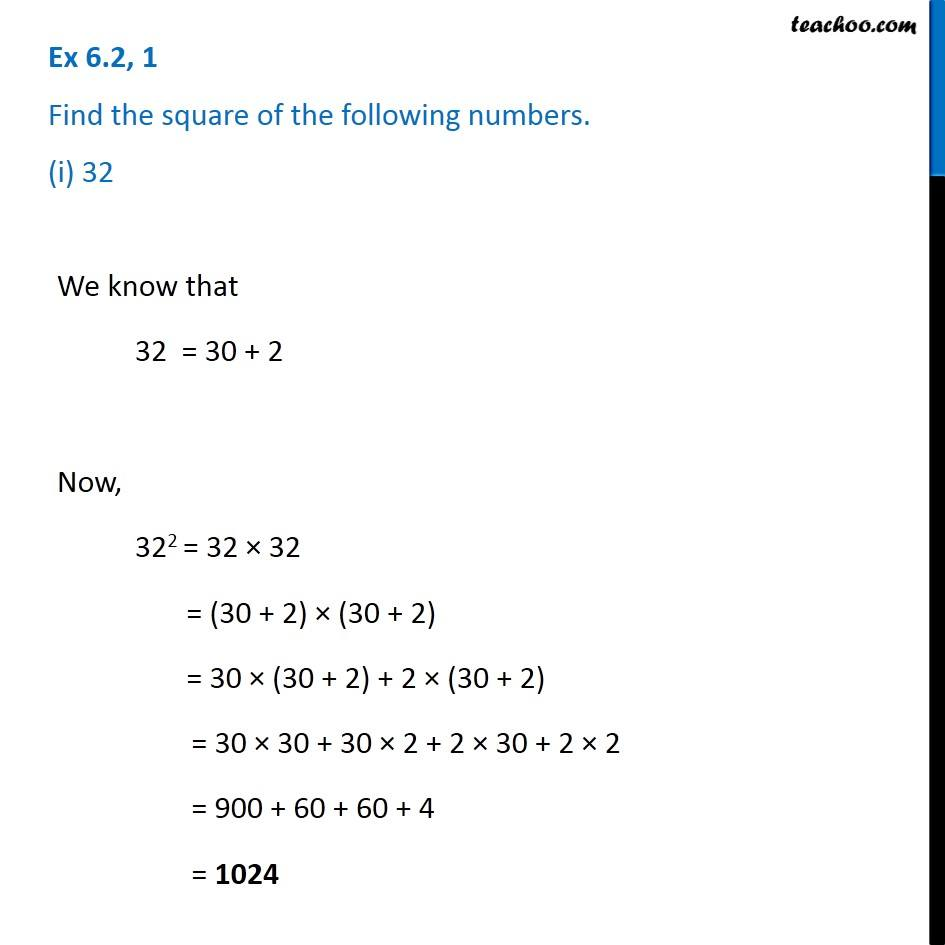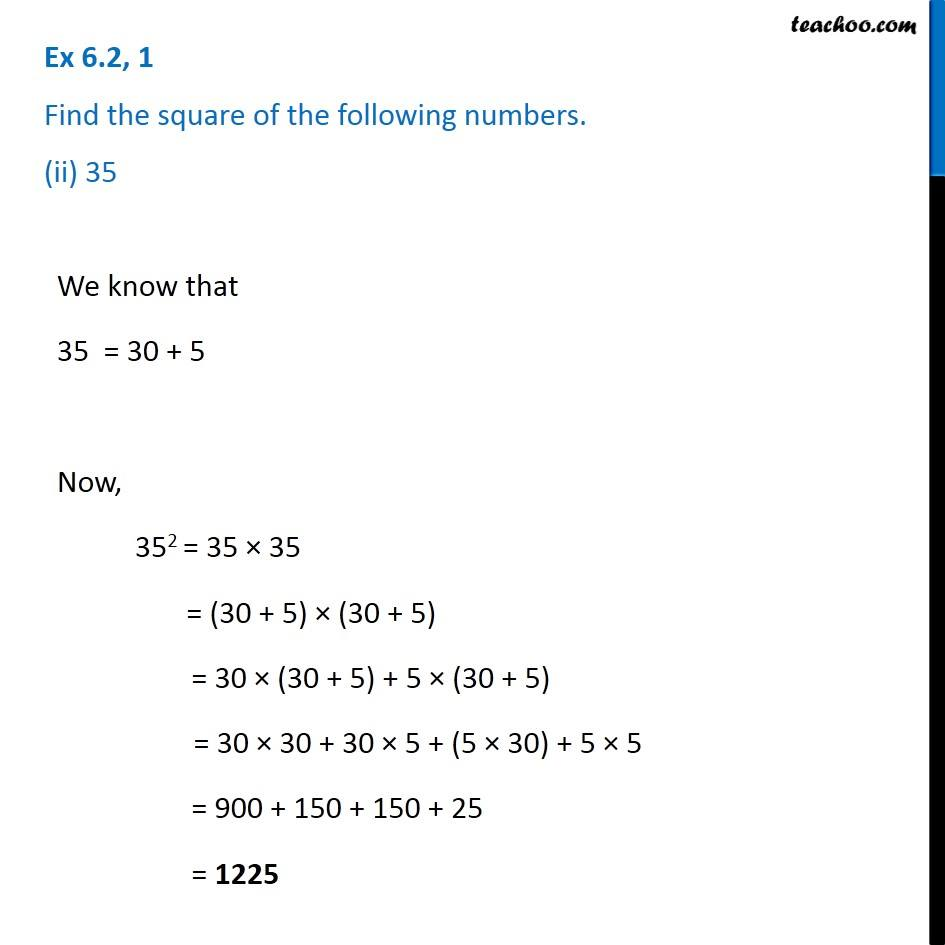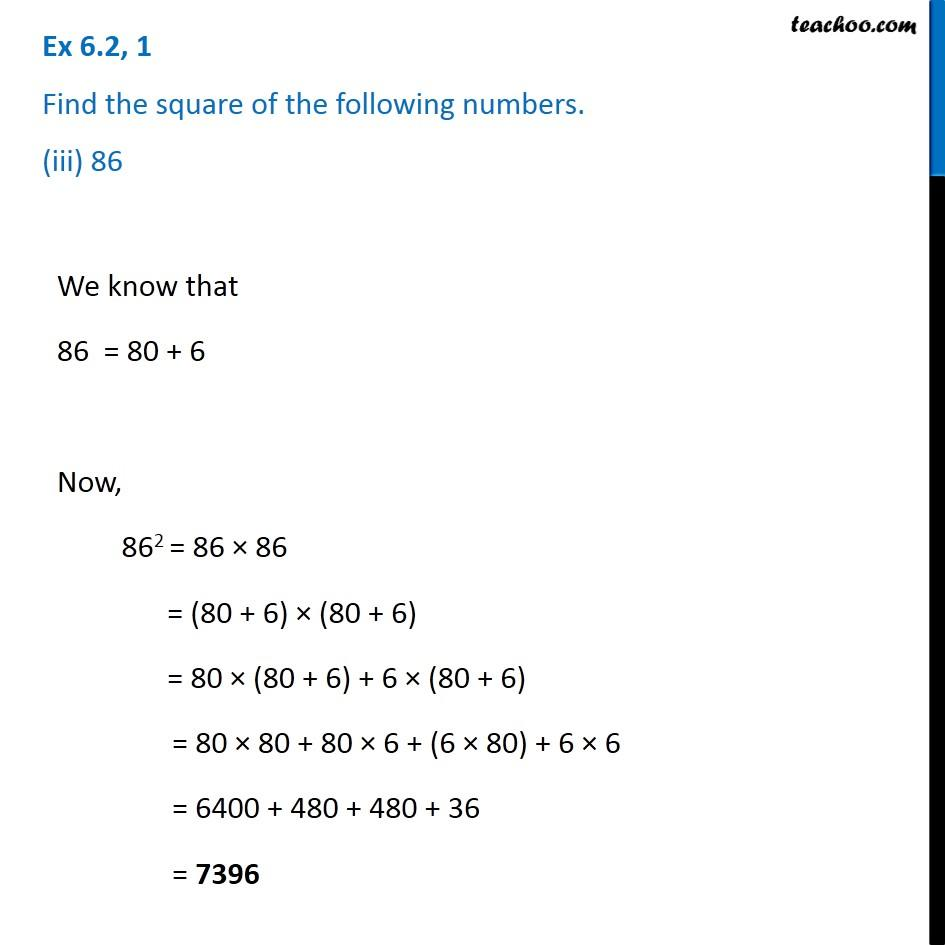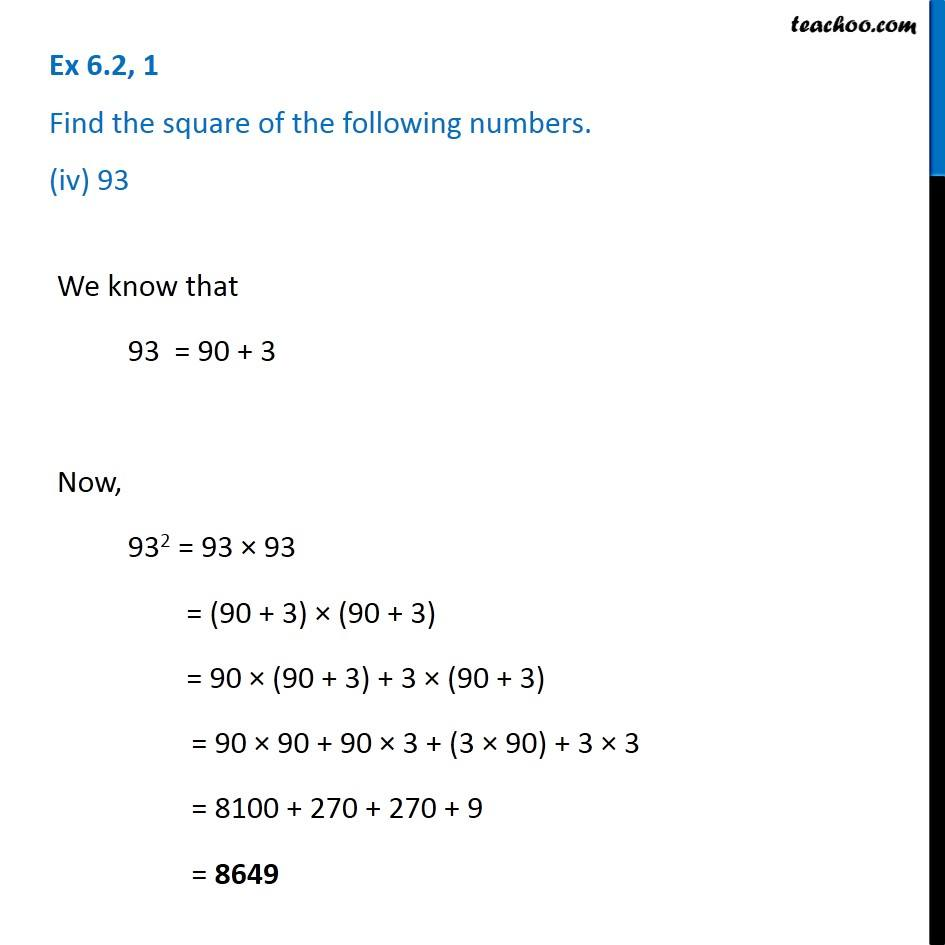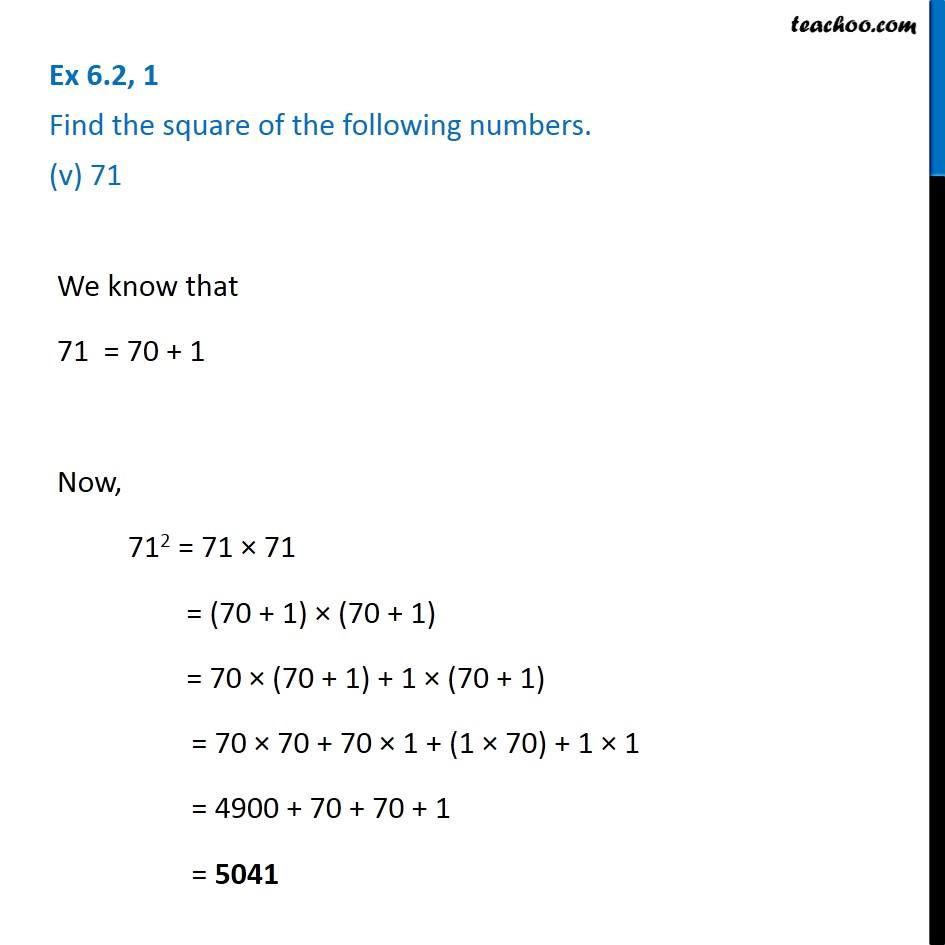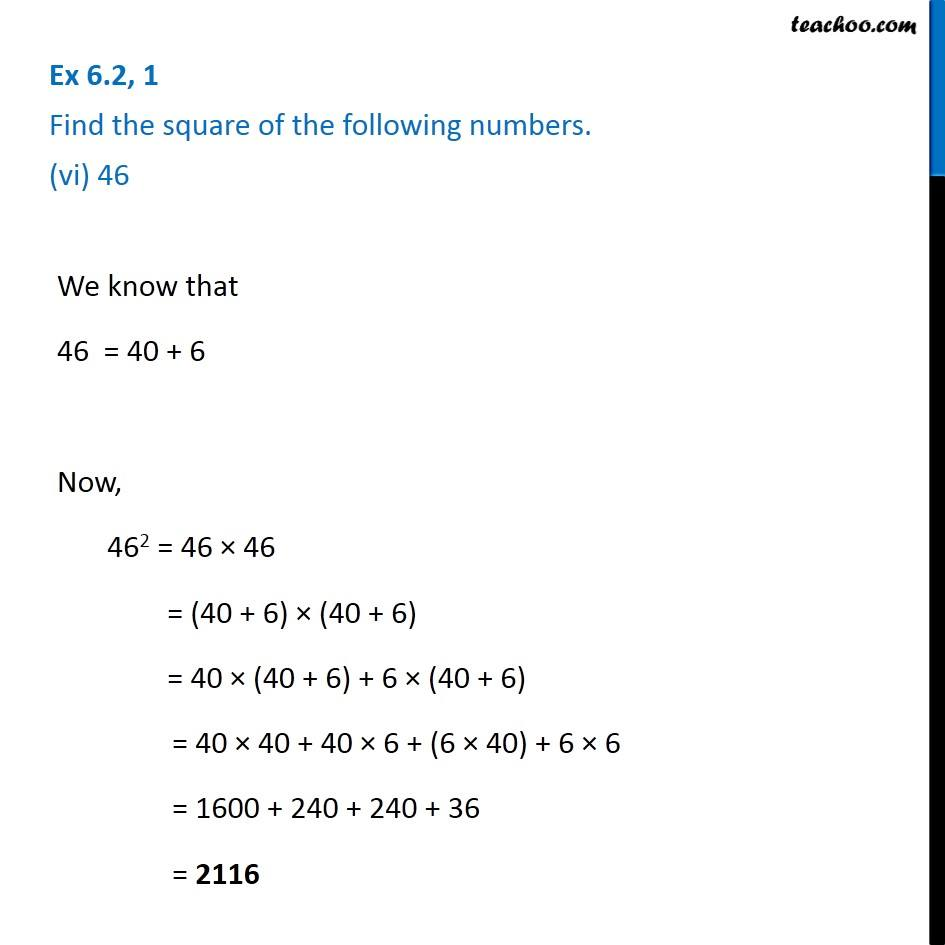Subscribe to our Youtube Channel - https://you.tube/teachoo

1. Chapter 6 Class 8 Squares and Square Roots
2. Serial order wise
3. Ex 6.2

Transcript

Ex 6.2, 1 Find the square of the following numbers. (i) 32We know that 32 = 30 + 2 Now, 322 = 32 × 32 = (30 + 2) × (30 + 2) = 30 × (30 + 2) + 2 × (30 + 2) = 30 × 30 + 30 × 2 + 2 × 30 + 2 × 2 = 900 + 60 + 60 + 4 = 1024 Ex 6.2, 1 Find the square of the following numbers. (ii) 35We know that 35 = 30 + 5 Now, 352 = 35 × 35 = (30 + 5) × (30 + 5) = 30 × (30 + 5) + 5 × (30 + 5) = 30 × 30 + 30 × 5 + (5 × 30) + 5 × 5 = 900 + 150 + 150 + 25 = 1225 Ex 6.2, 1 Find the square of the following numbers. (iii) 86We know that 86 = 80 + 6 Now, 862 = 86 × 86 = (80 + 6) × (80 + 6) = 80 × (80 + 6) + 6 × (80 + 6) = 80 × 80 + 80 × 6 + (6 × 80) + 6 × 6 = 6400 + 480 + 480 + 36 = 7396 Ex 6.2, 1 Find the square of the following numbers. (iv) 93We know that 93 = 90 + 3 Now, 932 = 93 × 93 = (90 + 3) × (90 + 3) = 90 × (90 + 3) + 3 × (90 + 3) = 90 × 90 + 90 × 3 + (3 × 90) + 3 × 3 = 8100 + 270 + 270 + 9 = 8649 Ex 6.2, 1 Find the square of the following numbers. (v) 71We know that 71 = 70 + 1 Now, 712 = 71 × 71 = (70 + 1) × (70 + 1) = 70 × (70 + 1) + 1 × (70 + 1) = 70 × 70 + 70 × 1 + (1 × 70) + 1 × 1 = 4900 + 70 + 70 + 1 = 5041 Ex 6.2, 1 Find the square of the following numbers. (vi) 46 We know that 46 = 40 + 6 Now, 462 = 46 × 46 = (40 + 6) × (40 + 6) = 40 × (40 + 6) + 6 × (40 + 6) = 40 × 40 + 40 × 6 + (6 × 40) + 6 × 6 = 1600 + 240 + 240 + 36 = 2116

Ex 6.2